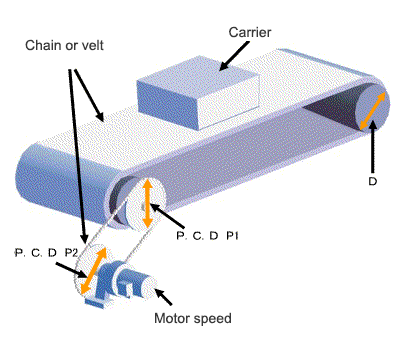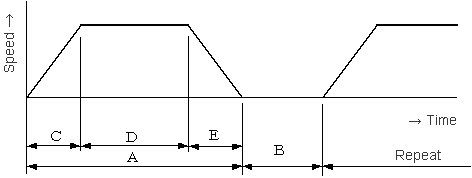# Technical Calculation of Power

## Gearmotor for chain conveyor driveIn the case of frequent start-stop operation, consider the load factor listed in a calalog.

### Summary of the machine

#### 1.1 Specification of machine

① Gross weight of the carrier
W = kg
② Gross weight of chain or velt
B = kg
P.C.D of main sprocket wheel, or Diameter of dram
D = mm
④ Frictional resistance
μ =

#### 1.2. Connecting method with reducer (gearmotor)

⑤ P.C.D of a sprocket wheel or a gear
P1 = mm
⑥ P.C.D of a sprocket wheel or a gear
P2 = mm

① Motor speed
N = r/min

### Decision of conveyance speed and reduction ratio

① Speed
V = m/min
②Reduction ratio (calculated value at ①)
Z =
① Reduction ratio
Z =
② Speed(calculated value at ①)
V = m/min

#### 4.1 Input of efficiency

① Efficiency of reducer and gearmotor
ηc =
② Efficiency of the sprocket wheel or the gear
ηg =

#### 4.2 Calculation result (load torque and motor power)

③ Load tprque (Converted in a motor shaft)
Tf = N･m
④Conversion from load torque Tf to motor-power Pf
Pf = kW

### Calculation of load moment of inertia

#### 5.1 Calculation result (load moment of inertia)

① Moment of inertia of carrier and chain or belt (Converted in a motor shaft)
JW = kg･m2

#### 5.2 The moment of inertia of total load

② Total weight of sprocket wheel and dram (Wd)
Wd = kg
③ Input the inside diameter D1=□mm in the case of the hollow type drum.
D1 = mm
④ Moment of inertia of sprocket wheel or drum P2 (Converted in a motor shaft)
jd = kg･m2
⑤ Total weight of sprocket wheel and gear (P1)
Wp1 = kg
⑥ Total weight of sprocket wheel and gear (P2)
Wp1 = kg
⑦ Moment of inertia of sprocket wheel or gear (Converted in a motor shaft)

Jp1 = kg･m2

Jp2 = kg･m2
② Moment of inertia of sprocket wheel or gear (Converted in a motor shaft)
Jp1+Jp2 = kg･m2
⑤ Calculating the moment of inertia of total load(Converted in a motor shaft)
Jf = kg･m2

### Temporary selection of motor

#### 6.1 Type of motor

① Motor power
kW × P
② Brake

③ Pattern of operation

### Pattern of operation

Commercial power supply
A = sec

B = secInverter control
C = sec

D = sec

E = sec

B = sec